ListConvolve

The next cell demonstrates the basic use of ListConvolve.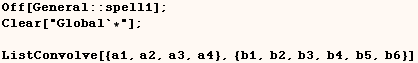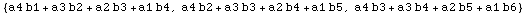Next we see that ListConvolve is equivalent to flattening the result of a
certain matrix product.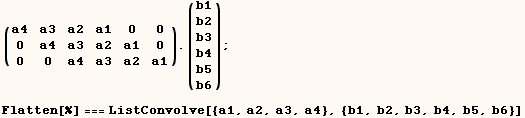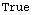The next cell shows that ListConvolve is closely related to ListCorrelate.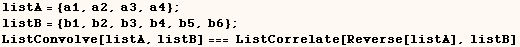Specifying the "overhang" using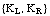In the next cell we provide ListConvolve {-1,-1} as a third argument.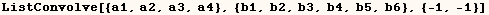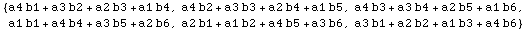The previous example is equivalent to the matrix product in the next cell.
Here we have the last element of {a1, a2, a3, a4} in the upper left position
of the left matrix..  We also have the last element of {a1, a2, a3, a4} in
the lower right position of the left matrix.  The (-1) indicates last element
of {a1, a2, a3, a4}, and (-2) would indicate the second from the last element
of {a1, a2, a3, a4}.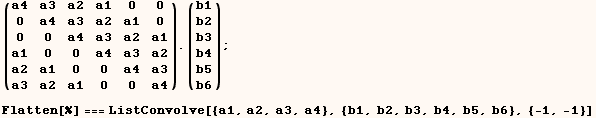In the next cell we provide ListConvolve {1,1} as a third argument.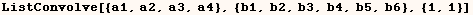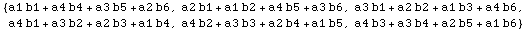The previous example is equivalent to the matrix product in the next cell.
Here we have the first element of {a1, a2, a3, a4} in the upper left position
of the left matrix..  We also have the first element of {a1, a2, a3, a4} in
the lower right position of the left matrix.  The (1) indicates first element
of {a1, a2, a3, a4}, and (2) would indicate the second element of {a1, a2,
a3, a4}.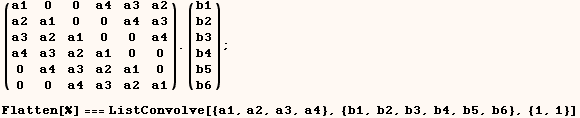In the next cell we provide ListConvolve {1,-1} as a third argument.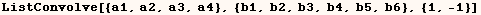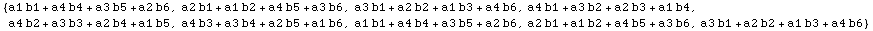The previous example is equivalent to the matrix product in the next cell.
Here we have the first element of {a1, a2, a3, a4} in the upper left position
of the left matrix..  We also have the last element of {a1, a2, a3, a4} in
the lower right position of the left matrix.  The (1) indicates first element
of {a1, a2, a3, a4}, and (-1) indicates the last element of {a1, a2, a3, a4}.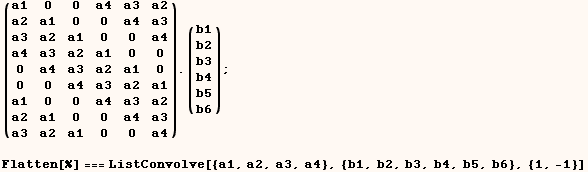In the next cell we provide ListConvolve {-1,1} as a third argument. Notice
this gives the same result as

ListConvolve[{a1,a2,a3,a4},{b1,b2,b3,b4,b5,b6}]  (ie. {-1,1} is the default
for the third argument).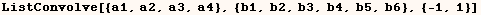The previous example is equivalent to the matrix product in the next cell.
Here we have the last element of {a1, a2, a3, a4} in the upper left position
of the left matrix..  We also have the First element of {a1, a2, a3, a4} in
the lower right position of the left matrix.  The (1) indicates first element
of {a1, a2, a3, a4}, and (-1) indicates the last element of {a1, a2, a3, a4}.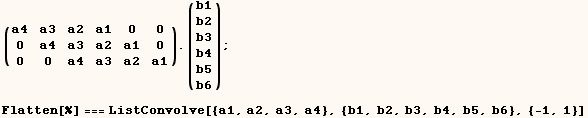In the next cell we provide (s) as a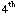argument.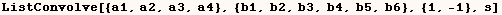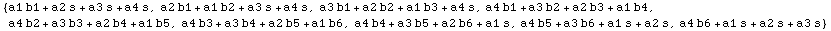The next cell performs a series of Dot products that give the same result as
in the previous cell.  Notice we gave ListConvolve {1,-1} as a third
argument, and the left part of the first row starts with the first part of
{a1,a2,a3,a4}.  Also the last row ends with the last element of
{a1,a2,a3,a4}.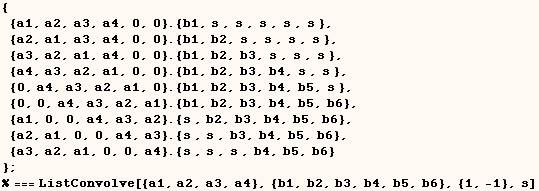In the next cell we provide {u1 ,u2, u3, u4, u5, u6} as aargument.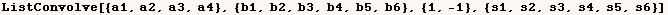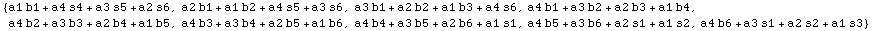The next cell performs a series of Dot products that give the same result  as in the previous cell. Notice the difference between giving (s) and giving  {s,t,u} as aargument.  I actually have a hard time seeing when this would be useful.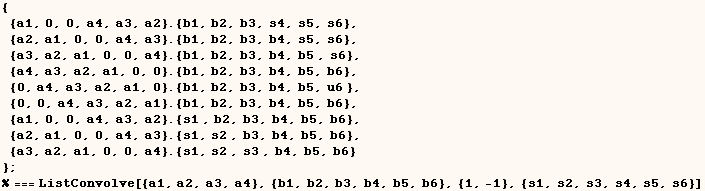To make this more clear I give another example where we give ListConvolve  aargument in the next cell.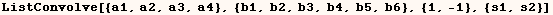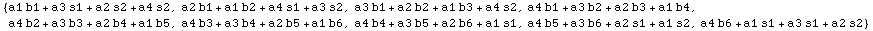The next cell performs a series of Dot products that give the same result as
in the previous cell.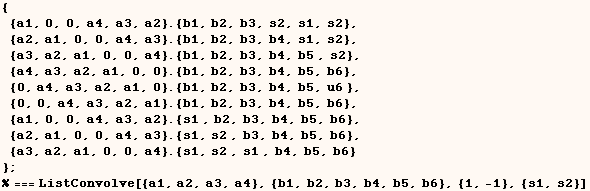It's curious that providing an empty list as aargument is equivalent to providing the integer 1 as aargument.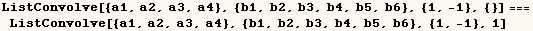The next cell shows that by default ListConvolve uses the second argument  in place of aargument when none is provided.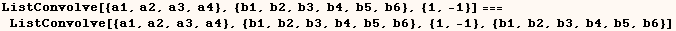Generalizing beyond Times, Plus

In the next cell we provide ListConvolve (g) as a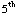argument and it uses the head (g) in place of Times.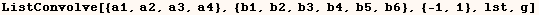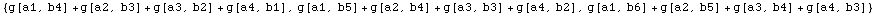In the next cell we provide ListConvolve (g, h) as aand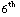arguments respectively and it uses the heads (g) and (h) in place of  Times and Plus respectively.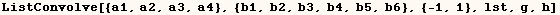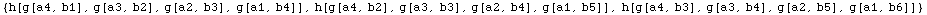ListConvolve with matrices

In the next cell we perform a ListConvolution on matrices.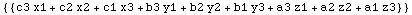The next cell shows how the same ListConvolution can be done as Dot products
on parts of the matrices.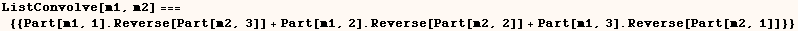We can give ListConvolve a level specification as a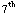argument.  The next cell shows that level (2) is the default  specification when working on matrices.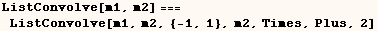In the next cell we give ListConvolve the integer (1) as a level
specification.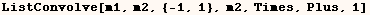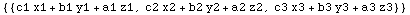The next cell shows how the same ListConvolution can be done as Dot products
on parts of the matrices.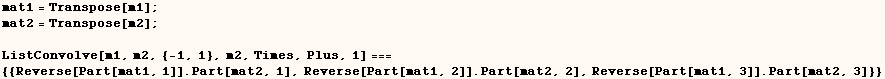The next cell shows two ways of expressing the default for the third argument
of ListConvolve when working with matrices.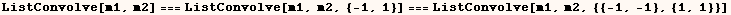I am having a hard time seeing a pattern to how a ListConvolution on matrices
is done in different cases.  I would like to have a complete understanding
for what the third argument of ListConvolve does when working with matrices,
but it's too hard to decipher.  For example I would like to understand the
result of the examples below.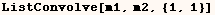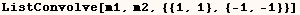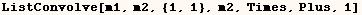I also understand we can also perform a ListConvolution on tensors, but that
is also too confusing for me to think about.

Created by Mathematica  (May 16, 2004)

Back to Ted’s Tricks index page